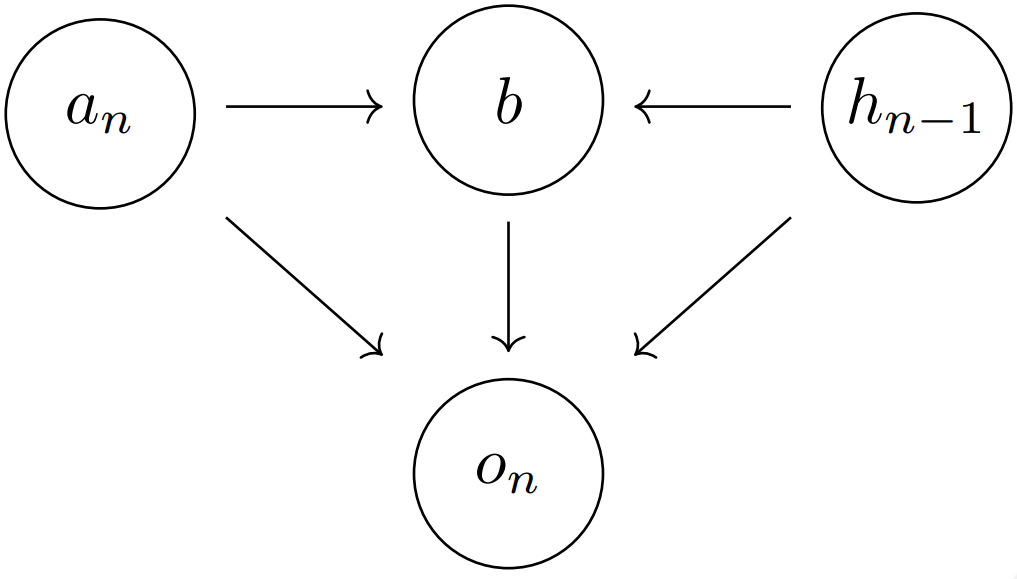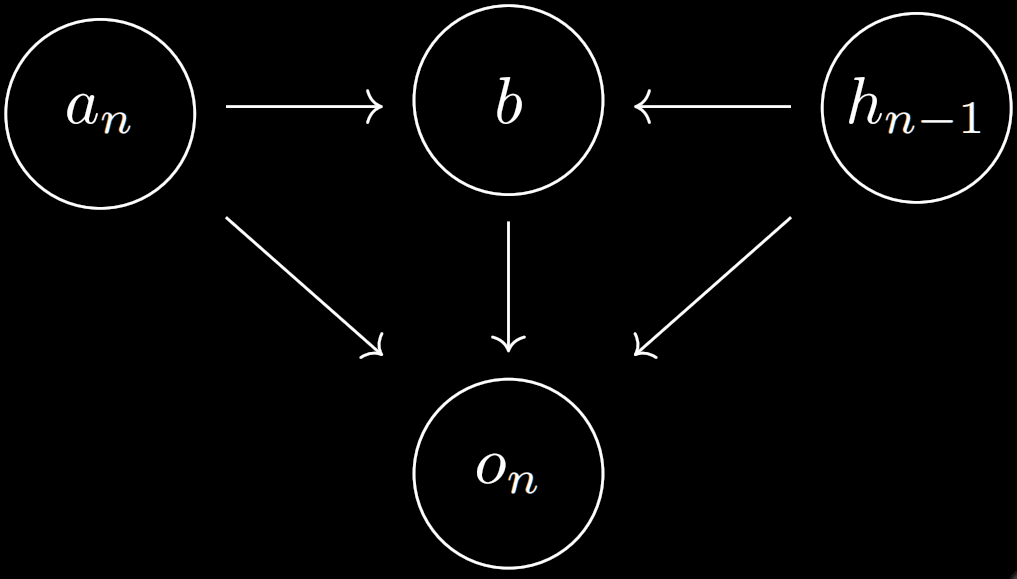# Oracles, sequence predictors, and self-confirming predictions

My counterfactual Oracle design uses a utility function/​reward function in order to train it to give the right predictions. Paul Christiano asked whether the whole utility function approach was necessary, and whether it could be replaced with a simple sequence predictor.

It turns out the answers are no, and yes. The counterfactual nature of the approach can be preserved, without needing a utility function.

# Counterfactual sequence prediction

There is a boxed Oracle, , which gets fed a series of observations , and must make a prediction about the next observation.

If the prediction was sent out into the world, then is attempting to make into a self-confirming prediction, with all the problems that this could entail.

However, we make into a counterfactual Oracle; on some occasions, the output is erased, and not seen by anyone. In that case, the Oracle will get a special message (for “erasure”), that will be added on after of .

Then the job of the counterfactual Oracle is, given a history (including past “”s), to produce a prediction that is the best prediction for given the history . In terms of words, the Oracle is trying to best predict the next observation, given that erasure is assumed to happen (the definition of “best” depends on the details of the design).

If our setup is well designed and there is no information leakage, then any has a probability that is independent of the prediction :

• .

## Meaning of predictions

If we ran the oracle without the assumption that would be observed, we’d have the standard self-confirming predictor (that being the only correct predictions); with that assumption, it becomes a counterfactual predictor.

Though counterfactuals are conceptually tricky, it’s actually easier to understand what a counterfactual predictor is doing than a general sequence predictor Oracle. We understand that a general sequence predictor is trying to find a self-confirming prediction—one where is the best estimate of , given - but unless we understand the structure of the world very well, we don’t have a clue where that self-confirming prediction might be.

In contrast, the counterfactual prediction is easy to interpret: “had we not seen , that is what would have been”; there are no loops in the definition.

## Extent of manipulation via prediction

Note that the Oracle can be used to estimate the extent to which the prediction is manipulative, by contrasting its predictions for given the , and given . This reveals the extent to which the prediction is potentially manipulative.

## Non-explicit erasure

If the Oracle isn’t given an explicit erasure message, but has a causal model of the world, we can still run the counterfactual Oracle by having it choose to be the best fit for the , conditional on and on the erasure happening.

# Factoring out certain routes

Assume now that the AI is an active AI, that can take actions in the world, affecting the observation . So we’ll replace terminology with ; note that the predictions were already actions, just actions of a particular type in particular setup.

We want to have the AI only consider certain routes to influence . This might be because there is a reward-function learning process involved, and we don’t want the AI to influence the learning process. Or maybe there is a corrigibility button involved, and we don’t want the AI to try and ensure it is pressed or not pressed.

In any case, label the banned routes of influence by the variables :Note that the Oracle case is just a special case of this, where corresponds to “humans reading the prediction”, and there being no direct arrow from to .

## Factoring out

Assume that is the default action. Then we want the AI to maximise some expected score of and , but acting as if the values of were set by rather than the value of .

Let be the possible values of . We require one more assumption: that if is possible given , then it is also possible given any other action (technically, we only need for the conditional probabilities to make sense).

Then if the AI has sufficient data to estimate expressions like , we act as if the AI was off-policy where is concerned. Namely, we get it to compute the action that maximises:

• .

Note that if we had instead of in that expression, then this would just be the classical .

This construction is essentially the same as the counterfactually uninfluenceable agent design, with representing the weights of the different reward functions that the AI was to “learn”.

No nominations.
No reviews.